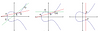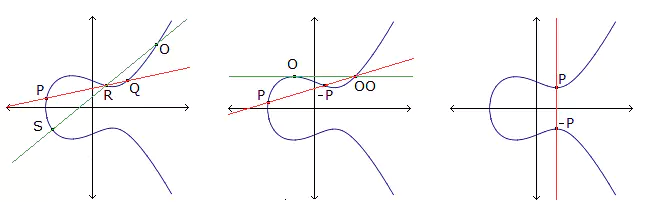# New Bounds for Linear Codes of Covering Radius 2

### Journal article

D. Bartoli, A. Davydov, M. Giulietti, S. Marcugini, F. Pambianco
ICMCTA, 2017

Semantic Scholar DBLP DOI
Cite

### Cite

APA
Bartoli, D., Davydov, A., Giulietti, M., Marcugini, S., & Pambianco, F. (2017). New Bounds for Linear Codes of Covering Radius 2. ICMCTA.

Chicago/Turabian
Bartoli, D., A. Davydov, M. Giulietti, S. Marcugini, and F. Pambianco. “New Bounds for Linear Codes of Covering Radius 2.” ICMCTA (2017).

MLA
Bartoli, D., et al. “New Bounds for Linear Codes of Covering Radius 2.” ICMCTA, 2017.

## Abstract

The length function (\ell _q(r,R)) is the smallest length of a q-ary linear code of covering radius R and codimension r. New upper bounds on (\ell _q(r,2)) are obtained for odd (r\ge 3). In particular, using the one-to-one correspondence between linear codes of covering radius 2 and saturating sets in the projective planes over finite fields, we prove that

\begin{aligned} \ell _q(3,2)\le \sqrt{q(3\ln q+\ln \ln q)}+\sqrt{\frac{q}{3\ln q}}+3 \end{aligned}

and then obtain estimations of (\ell _q(r,2)) for all odd (r\ge 5). The new upper bounds are smaller than the previously known ones. Also, the new bounds hold for all q, not necessary large, whereas the previously best known estimations are proved only for q large enough.

Share